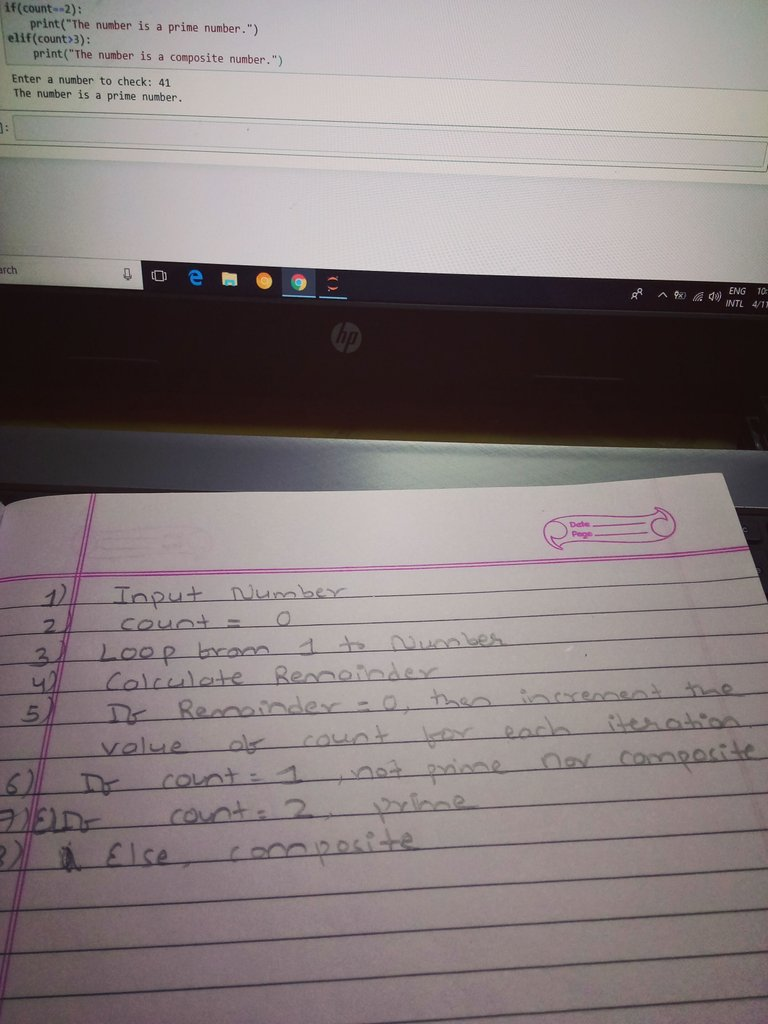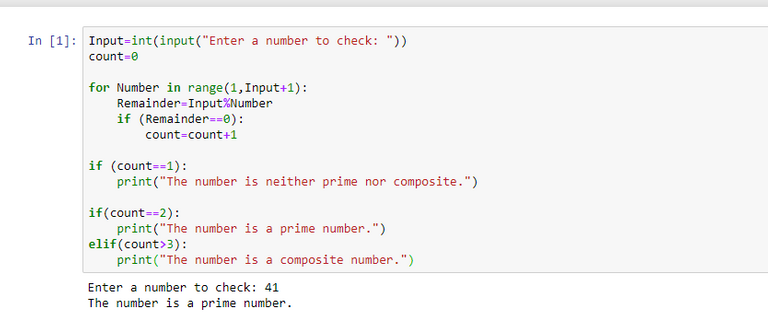# PYTHON PROGRAM TO CHECK A NUMBER FOR PRIME OR COMPOSITE!!

in GEMS2 years ago

I bet everyone know what is prime number and composite number. Prime numbers are those which have factors 1 and itself. Whereas, composite numbers are those having factors besides 1 and itself. This is the concept which I used to program for the python. I didn't use the help or tutorial of any online learning sites or youtube to code. I wrote down the pseudocode myself and then try to code in python. I hope you all guys can do the same. First learn and practice and practice. If it doesn't help at last then seek the help or other options. This was my simple pseudocode.``````Input=int(input("Enter a number to check: "))
count=0

for Number in range(1,Input+1):
Remainder=Input%Number
if (Remainder==0):
count=count+1

if (count==1):
print("The number is neither prime nor composite.")

if(count==2):
print("The number is a prime number.")

elif(count>=3):
print("The number is a composite number.")

``````

The output as executed by me is as follows. I could successfully run the code and display correct output at the first attempt.Run this code online here.

Sort:
UpvoteBankThis post have been upvoted by the @UpvoteBank service. Want to know more and receive "free" upvotes click here